# Calculus 3 : Divergence Theorem

## Example Questions

1 3 Next →

### Example Question #21 : Divergence Theorem

Find the divergence of the function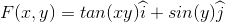at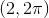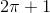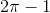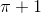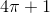Explanation:

Divergence can be viewed as a measure of the magnitude of a vector field's source or sink at a given point.

To visualize this, picture an open drain in a tub full of water; this drain may represent a 'sink,' and all of the velocities at each specific point in the tub represent the vector field. Close to the drain, the velocity will be greater than a spot farther away from the drain.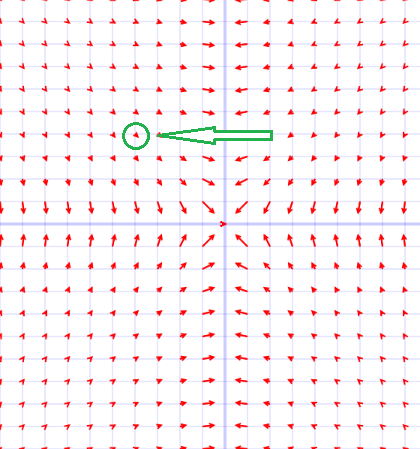What divergence can calculate is what this velocity is at a given point. Again, the magnitude of the vector field.

We're given the functionatWhat we will do is take the derivative of each vector element with respect to its variable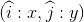Then sum the results together:

Trigonometric derivative: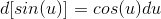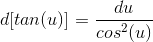Note that u may represent large functions, and not just individual variables!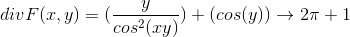### Example Question #22 : Divergence Theorem

Find the divergence of the function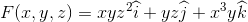at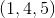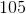Explanation:

Divergence can be viewed as a measure of the magnitude of a vector field's source or sink at a given point.

To visualize this, picture an open drain in a tub full of water; this drain may represent a 'sink,' and all of the velocities at each specific point in the tub represent the vector field. Close to the drain, the velocity will be greater than a spot farther away from the drain.What divergence can calculate is what this velocity is at a given point. Again, the magnitude of the vector field.

We're given the functionatWhat we will do is take the derivative of each vector element with respect to its variable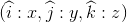Then sum the results together: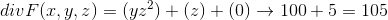### Example Question #23 : Divergence Theorem

Find the divergence of the function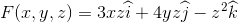at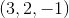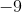Explanation:

Divergence can be viewed as a measure of the magnitude of a vector field's source or sink at a given point.

To visualize this, picture an open drain in a tub full of water; this drain may represent a 'sink,' and all of the velocities at each specific point in the tub represent the vector field. Close to the drain, the velocity will be greater than a spot farther away from the drain.What divergence can calculate is what this velocity is at a given point. Again, the magnitude of the vector field.

We're given the functionatWhat we will do is take the derivative of each vector element with respect to its variableThen sum the results together: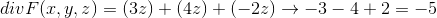### Example Question #24 : Divergence Theorem

Find the divergence of the function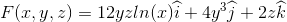at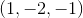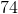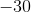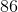Explanation:

Divergence can be viewed as a measure of the magnitude of a vector field's source or sink at a given point.

To visualize this, picture an open drain in a tub full of water; this drain may represent a 'sink,' and all of the velocities at each specific point in the tub represent the vector field. Close to the drain, the velocity will be greater than a spot farther away from the drain.What divergence can calculate is what this velocity is at a given point. Again, the magnitude of the vector field.

We're given the functionatWhat we will do is take the derivative of each vector element with respect to its variableThen sum the results together:

Derivative of a natural log: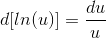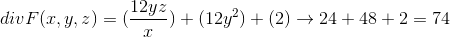1 3 Next →